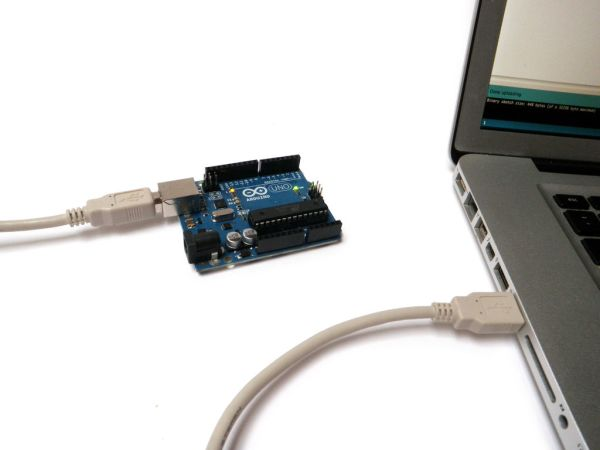Published: August 01, 2014, Edited by: Mads Hobye

# Communicate between Arduino and ProcessingGetting simple communication between Arduino and Processing can be tricky. This little solution solves the problem.

It is based on the easytransfer protocol. Behind the scenes the system syncronises a struct (dataset) between the computer and the arduino board.

### Limitations:

The data structure can only be int on arduino and short in processing. This is a consequence of a bug in the communication format (little indian to big indian conversion).

### Get it up and running

• Copy "EasyTransferProcessing" to your processing library (documents/processing/libraries).
• Copy "EasyTransferArduino" to your arduino library (documents/arduino/libraries).
• In Processing open the example: EasyTransferBasicCom.
• In Arduino open the example: EasyTransfer_Example.
• Start the program in Processing.

You will need to change the serial port in the processing code to the port number the Arduino board is connected to:

``````String portName = Serial.list();
``````

Communication is based on a struct with param, value. The param represents what to do and the value the value of the param.

`````` if (et_receive())
{
if (data.param==2)
{
background((data.value+20)*10, 0, 0);
}
}
``````

Similarly on the Arduino side:

``````mydata.param = 2;
mydata.value = (value ++ ) % 250;

//send the data
ET.sendData();
``````

### Alternatives:

The most common alternative is the firmata protocol: http://firmata.org/wiki/Main_Page